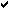# * Astronomy

 Home -> Astronomy News -> Forum help -> Standard deviation
Post Info TOPIC: Standard deviationLPosts: 131433
Date:
Standard deviation

CI(nSigma) = erf(n/sqrt(2))

 Sigma Confidence Interval 1 0.6826894850 2 0.9544997241 3 0.9973002038 4 0.9999366575 5 0.9999994267 6 0.9999999980

__________________LPosts: 131433
Date:
 Permalink Standard deviation, or lower case sigma, measures the spread, or deviation, of data about a central tendency, such as the mean or the median in a data set or group, with a Gaussian (bell curve) distribution.Standard deviation is a widely used measure of variability or diversity used in statistics and probability theory. It shows how much variation or "dispersion" exists from the average (mean, or expected value). A low standard deviation indicates that the data points tend to be very close to the mean, whereas high standard deviation indicates that the data points are spread out over a large range of values.Read more   __________________
 Page 1 of 1  sorted by Oldest FirstNewest First